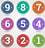# Number SystemQuantitative Aptitude Questions and Answers section on “Number-system” with solution and explanation for competitive examinations such as CAT, MBA, SSC, Bank PO, Bank Clerical and other examinations.

# A number when divided by 296 gives a remainder 75. When the same number is divided by 37, the remainder will be :

A number when divided by 296 gives a remainder 75. When the same number is divided by 37, the remainder will be : [A]1 [B]2 [C]8 [D]11 Show Answer 1 Let number be x. ∴ x = 296 Q + 75 where Q is the quotient and can have the value 1, 2, 3 etc. ..

# How many natural numbers divisible by 7 are there between 3 and 200?

How many natural numbers divisible by 7 are there between 3 and 200? [A]27 [B]28 [C]29 [D]36 Show Answer 28 Number just greater than 3 which is divisible by 7 = 7 Number just smaller than 200 which is divisible by 7 = 196 Here, a = 7, an = 196, d = 7, n ..

# Aptitude Question ID: 106413

If of the difference of and is subtracted from of the result is : [A] [B] [C] [D] Show Answer Hence option [D] is right answer.

# The product of two number is 9375 and the quotient, when the larger one is divided by the smaller, is 15. The sum of the numbers is :

The product of two number is 9375 and the quotient, when the larger one is divided by the smaller, is 15. The sum of the numbers is : [A]380 [B]395 [C]400 [D]425 Show Answer 400 Let the numbers be x and y and x is greater than y. As given, xy = 9375 …….. (I) ..

# Each number of a picnic party contributed twice as many rupees as the total number of members and the total collection was 3042 Rs. The number of members present in the party was :

Each member of a picnic party contributed twice as many rupees as the total number of members and the total collection was 3042 Rs. The number of members present in the party was : [A]22 [B]32 [C]39 [D]40 Show Answer 39 Let the required number of persons be x. According to the question, Hence option ..